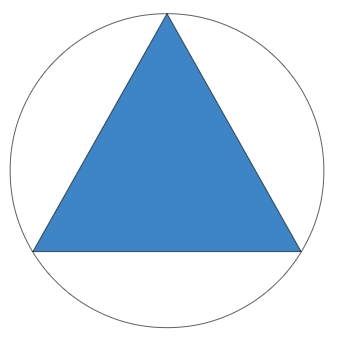# Program to calculate area of Circumcircle of an Equilateral Triangle in C++

As the name suggests, equilateral triangle is the one that have equal sides and also it have equal interior angles of 60° each. It is also known as regular triangle because it’s a regular polygon

Properties of equilateral triangle are

• 3 sides of equal length
• Interior angles of same degree which is 60

Circumcircle of a plygon is the circle that passes through all the vertices of a polygon. The radius of circle can be an edge or side of polygon inside the circle which is known as circumradius and center of circle is known as circumcenter. It can be inside or outside of the circle

Given below is the figure of Circumcircle of an Equilateral triangle## Problem

Given with the side of equilateral triangle the task is to find the area of a circumcircle of an equilateral triangle where area is the space occupied by the shape.

To calculate area of circumcircle of an equilateral triangle there is a formula −

Area = (π*a^2)/3

## Example

Input-: a = 5.0
Output-: Area of CircumCircle of equilateral triangle is :26.1667

## Algorithm

Start
Step 1 -> define macro for pi value
#define pi 3.14
Step 2 -> declare function to calculate area of circumcircle of equilateral triangle
float area_circum(float a)
return (a * a * (pi / 3))
Step 3 -> In main()
Declare variables as float a, area
Set a = 5
Set area = area_circum(a)
Print area
Stop

## Example

#include <iostream>
#include <math.h>
#define pi 3.14
using namespace std;
// function to calculate the area of circumcircle of equilateral triangle
float area_circum(float a){
return (a * a * (pi / 3));
}
int main(){
float a, area;
a = 5.0;
area = area_circum(a);
cout << "Area of CircumCircle of equilateral triangle is :" <<area;
return 0;
}

## Output

Area of CircumCircle of equilateral triangle is :26.1667

Updated on: 20-Sep-2019

92 Views